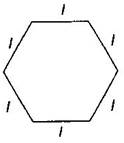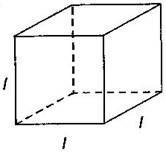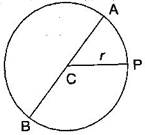1. /
2. CBSE
3. /
4. Class 06
5. /
6. Mathematics
7. /
8. NCERT Solutions for Class...

# NCERT Solutions for Class 6 Maths Exercise 11.2

NCERT solutions for Maths Algebra## NCERT Solutions for Class 6 Maths Algebra

###### Question 1.The side of an equilateral triangle is shown by {tex}l.{/tex} Express the perimeter of the equilateral triangle using {tex}l.{/tex}

Answer: Side of equilateral triangle = {tex}l{/tex}

Therefore, Perimeter of equilateral triangle = 3 x side = {tex}3l{/tex}

###### Question 2.The side of a regular hexagon is denoted by {tex}l.{/tex} Express the perimeter of the hexagon using {tex}l.{/tex} (Hint: A regular hexagon has all its six sides in length)Answer: Side of hexagon = {tex}l{/tex}

Therefore, Perimeter of Hexagon = 6 x side = {tex}6l{/tex}

NCERT Solutions for Class 6 Maths Exercise 11.2

###### Question 3.A cube is a three-dimensional figure. It has six faces and all of them are identical squares. The length of an edge of the cube is given by {tex}l.{/tex} find the formula for the total length of the edges of a cube.Answer: Length of one edge of cube = {tex}l{/tex}

Number of edges in a cube = 12

Therefore, total length = {tex}12 \times l = 12l{/tex}

###### Question 4.The diameter of a circle is a line, which joins two points on the circle and also passes through the centre of the circle. (In the adjoining figure AB is a diameter of the circle; C is its centre). Express the diameter of the circle {tex}\left( d \right){/tex} in terms of its radius {tex}\left( r \right).{/tex}Therefore, {tex}d = 2r{/tex}

NCERT Solutions for Class 6 Maths Exercise 11.2

###### Question 5.To find sum of three numbers 14, 27 and 13. We can have two ways.

(a)We may first add 14 and 27 to get 41 and then add 13 to it to get the total sum 54, or

(b)We may add 27 and 13 to get 40 and then add 14 to get the sum 54. Thus

(14 + 27) + 13 = 14 + (27 + 13)

This can be done for any three numbers. This property is known as the associativity of addition of numbers. Express this property which we have already studied in the chapter on Whole Numebrs, in a general way, by using variables {tex}a,b{/tex} and {tex}c.{/tex}

Answer: {tex}\left( {a + b} \right) + c = a + \left( {b + c} \right){/tex}

## NCERT Solutions for Class 6 Maths Exercise 11.2

NCERT Solutions Class 6 Maths PDF (Download) Free from myCBSEguide app and myCBSEguide website. Ncert solution class 6 Maths includes text book solutions from Class 6 Maths Book . NCERT Solutions for CBSE Class 6 Maths have total 14 chapters. 6 Maths NCERT Solutions in PDF for free Download on our website. Ncert Maths class 6 solutions PDF and Maths ncert class 6 PDF solutions with latest modifications and as per the latest CBSE syllabus are only available in myCBSEguide.

## CBSE app for Students

To download NCERT Solutions for Class 6 Maths, Social Science Computer Science, Home Science, Hindi English, Maths Science do check myCBSEguide app or website. myCBSEguide provides sample papers with solution, test papers for chapter-wise practice, NCERT solutions, NCERT Exemplar solutions, quick revision notes for ready reference, CBSE guess papers and CBSE important question papers. Sample Paper all are made available through the best app for CBSE students and myCBSEguide website.Test Generator

Create question paper PDF and online tests with your own name & logo in minutes.myCBSEguide

Question Bank, Mock Tests, Exam Papers, NCERT Solutions, Sample Papers, Notes